×#### Thank you for registering.

One of our academic counsellors will contact you within 1 working day.

Click to Chat

1800-1023-196

+91-120-4616500

CART 0

• 0

MY CART (5)

Use Coupon: CART20 and get 20% off on all online Study Material

ITEM
DETAILS
MRP
DISCOUNT
FINAL PRICE
Total Price: Rs.

There are no items in this cart.
Continue Shopping• Complete JEE Main/Advanced Course and Test Series
• OFFERED PRICE: Rs. 15,900
• View Details

```Chapter 14: Compound Interest Exercise – 14.2

Question: 1

Compute the amount and the compound interest in each of the following by using the formulae when:

(i) Principal = Rs 3000, Rate = 5%, Time = 2 years

(ii) Principal = Rs 3000, Rate =18%, Time = 2 years

(iii) Principal = Rs 5000, Rate = 10 paise per rupee per annum, Time = 2 years

(iv) Principal = Rs 2000, Rate = 4 paise per rupee per annum, Time = 3 years

(v) Principal = Rs 12800, Rate = 7(1/2)%, Time = 3 years

(vi) Principal =Rs 10000, Rate 20% per annum compounded half-yearly, Time = 2 years

(vii) Principal = Rs 160000, Rate =10 paise per rupee per annum compounded half-yearly, Time = 2 years.

Solution: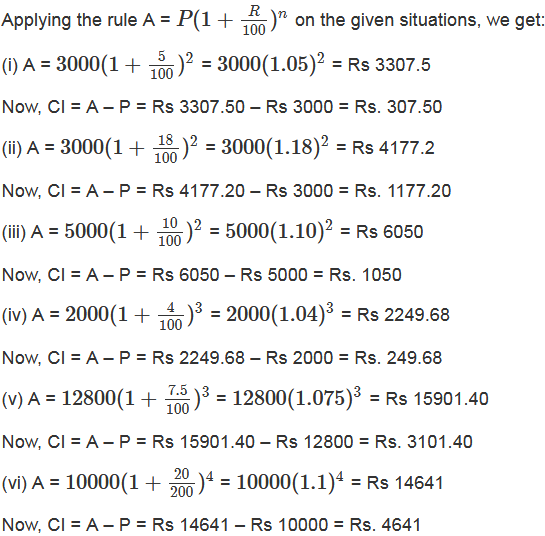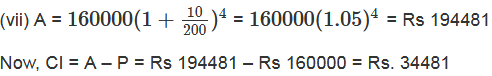Question: 2

Find the amount of Rs 2400 after 3 years, when the interest is compounded annually at the rate of 20% per annum.

Solution:

Given:

P = Rs 2400

R = 20 % p. a

n = 3 years

We know that amount A at the end of n years at the rate R% per annum when the interest is compounded annually is given byQuestion: 3

Rahman lent Rs 16000 to Rasheed at the rate of 12(1/2)% per annum compound interest. Find the amount payable by Rasheed to Rahman after 3 years.

Solution:

Given:

P = Rs 16000

R = 12.5 % p.a

n = 3 years

We know that amount A at the end of n years at the rate R% per annum when the interest is compounded annually is given byQuestion: 4

Meera borrowed a sum of Rs 1000 from Sita for two years. If the rate of interest is 10% compounded annually, find the amount that Meera has to pay back.

Solution:

Given:

P = Rs 1000

R = 10 % p.a

n = 2 years

We know that amount A at the end of n years at the rate R% per annum when the interest is compounded annually is given by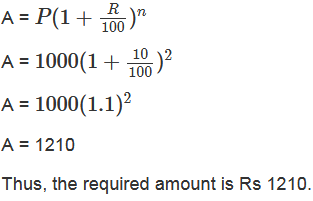Question: 5

Find the difference between the compound interest and simple interest. On a sum of Rs 50,000 at 10% per annum for 2 years.

Solution:

Given:

P = Rs 50000

R = 10 % p. a

n = 2 years

We know that amount A at the end of n years at the rate R% per annum when the interest is compounded annually is given byQuestion: 6

Amit borrowed Rs 16000 at 17(1/2)% per annum simple interest. On the same day, he lent it to Ashu at the same rate but compounded annually. What does he gain at the end of 2 years?

Solution: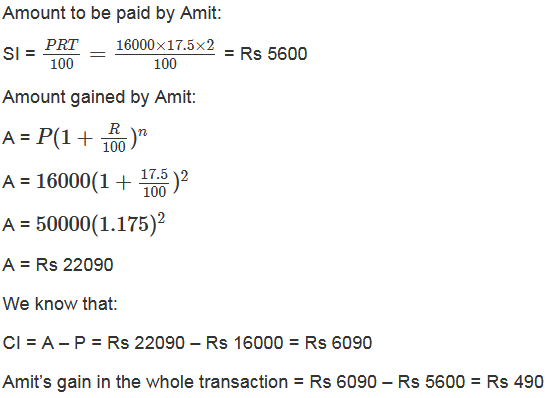Question: 7

Find the amount of Rs 4096 for 18 months at 12(1/2)% per annum, the interest being compounded semi-annually.

Solution:

Given:

P = Rs 4096

R = 12.5 % p. a

n = 1.5 years

We have: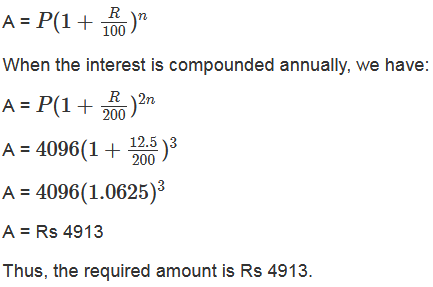Question: 8

Find the amount and the compound interest on Rs 8000 for 1(1/2) years at 10% per annum, compounded half-yearly.

Solution:Question: 9

Kamal borrowed Rs 57600 from LIC against her policy at 12(1/2)% per annum to build a house. Find the amount that she pays to the LIC after 1(1/2) years if the interest is calculated half-yearly.

Solution:Question: 10

Abha purchased a house from Avas Parishad on credit. If the cost of the house is Rs 64000 and the rate of interest is 5% per annum compounded half-yearly, find the interest paid by Abha after one year and a half.

Solution: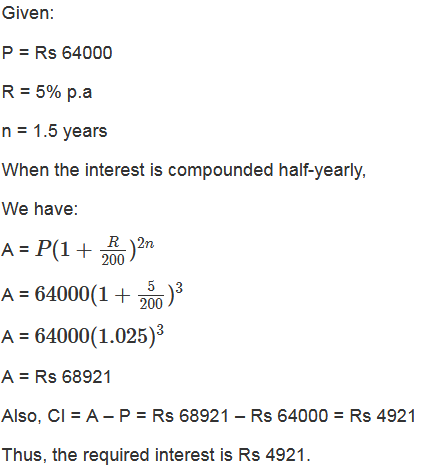Question: 11

Rakesh lent out Rs 10000 for 2 years at 20% per annum, compounded annually. How much more he could earn if the interest be compounded half-yearly?

Solution: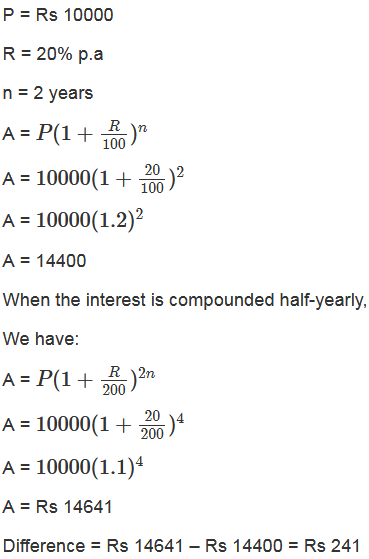Question: 12

Romesh borrowed a sum of Rs 245760 at 12.5% per annum, compounded annually. On the same day, he lent out his money to Ramu at the same rate of interest, but compounded semi-annually. Find his gain after 2 years.

Solution:

Given:

P = Rs 245760

R = 12.5% p.a

n = 2 years

When compounded annually,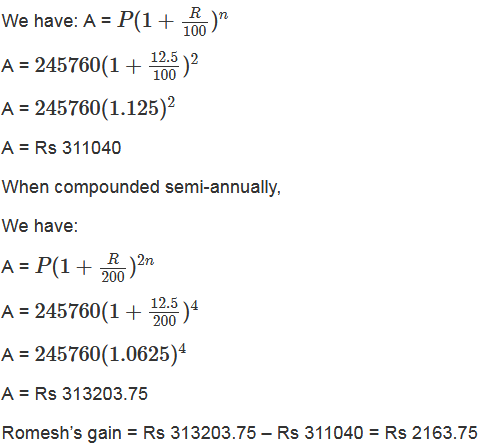Question: 13

Find the amount that David would receive if he invests Rs 8192 for 18 months at 12(1/2)% per annum, the interest being compounded half-yearly.

Solution: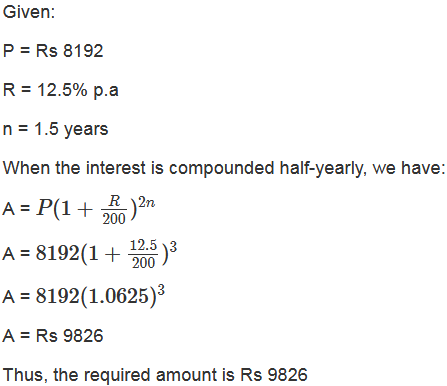Question: 14

Find the compound interest on Rs 15625 for 9 months, at 16% per annum, compounded quarterly.

Solution:Question: 15

Rekha deposited Rs 16000 in a foreign bank which pays interest at the rate of 20% per annum compounded quarterly, find the interest received by Rekha after one year.

Solution: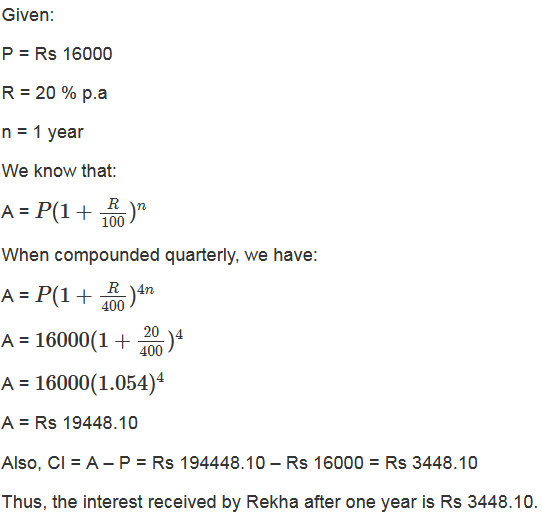Question: 16

Find the amount of Rs 12500 for 2 years compounded annually, the rate of interest being 15% for the first year and 16% for the second year.

Solution: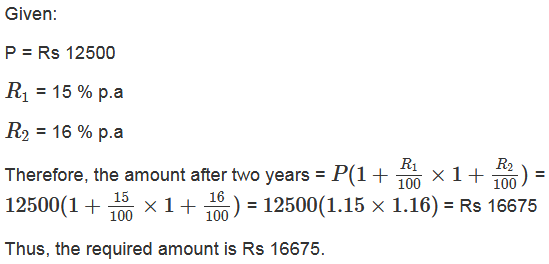Question: 17

Ramu borrowed Rs 15625 from a finance company to buy a scooter. If the rate of interest be 16% per annum compounded annually, what payment will he have to make after 2(1/4) years?

Solution:Question: 18

What will Rs 125000 amount to at the rate of 6%, if the interest is calculated after every four months?

Solution: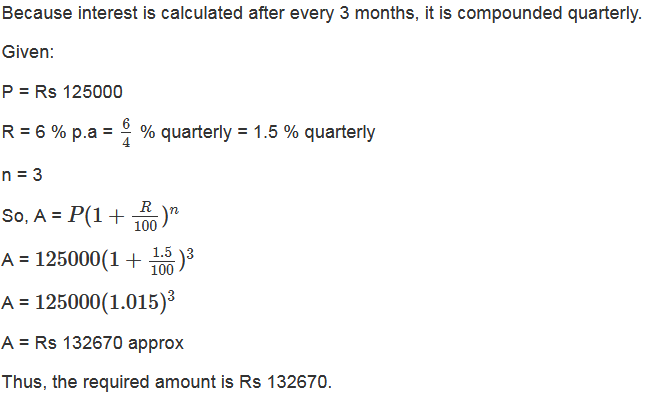Question: 19

Find the compound interest at the rate of 5% for three years on that principle which in three years at the rate of 5% per annum gives Rs 12000 as simple interest.

Solution:Question: 20

A sum of money was lent for 2 years at 20% compounded annually. If the interest is payable half-yearly instead of yearly, then the interest is Rs 482 more. Find the sum.

Solution: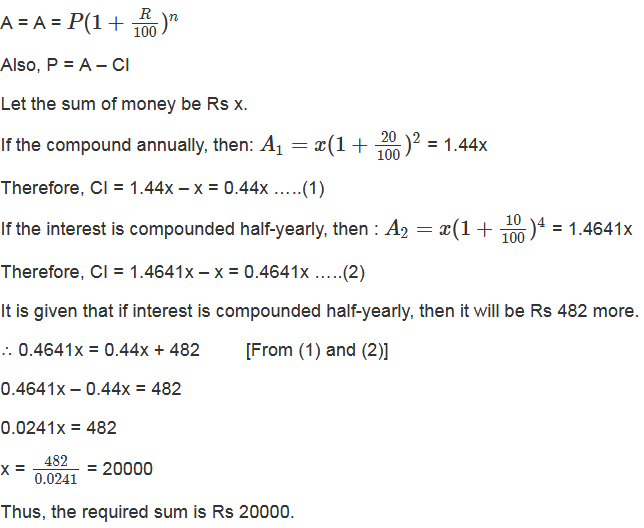Question: 21

Simple interest on a sum of money for 2 years at 6(1/2)% per annum is Rs 5200. What will be the compound interest on the sum at the same rate for the same period?

Solution: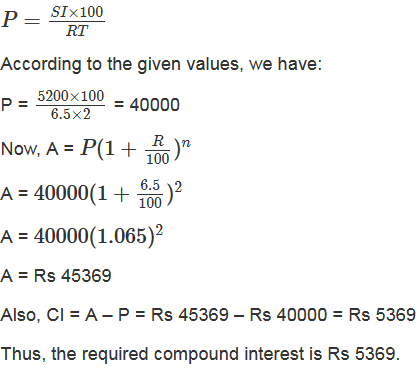Question: 22

Find the compound interest at the rate of 5% per annum for 3 years on that principle which in 3 years at the rate of 5% per annum gives Rs 1200 as simple interest.

Solution:```### Course Features

• 728 Video Lectures
• Revision Notes
• Previous Year Papers
• Mind Map
• Study Planner
• NCERT Solutions
• Discussion Forum
• Test paper with Video Solution